Main Content

# Visualize Activations of a Convolutional Neural Network

This example shows how to feed an image to a convolutional neural network and display the activations of different layers of the network. Examine the activations and discover which features the network learns by comparing areas of activation with the original image. Find out that channels in earlier layers learn simple features like color and edges, while channels in the deeper layers learn complex features like eyes. Identifying features in this way can help you understand what the network has learned.

The example requires Deep Learning Toolbox™ and the Image Processing Toolbox™.

### Load Pretrained Network and Data

Load a pretrained SqueezeNet network.

`net = squeezenet;`

Read and show an image. Save its size for future use.

```im = imread('face.jpg'); imshow(im)```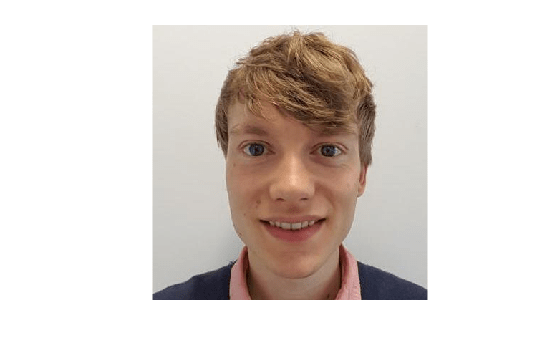```imgSize = size(im); imgSize = imgSize(1:2);```

### View Network Architecture

Analyze the network to see which layers you can look at. The convolutional layers perform convolutions with learnable parameters. The network learns to identify useful features, often with one feature per channel. Observe that the first convolutional layer has 64 channels.

`analyzeNetwork(net)`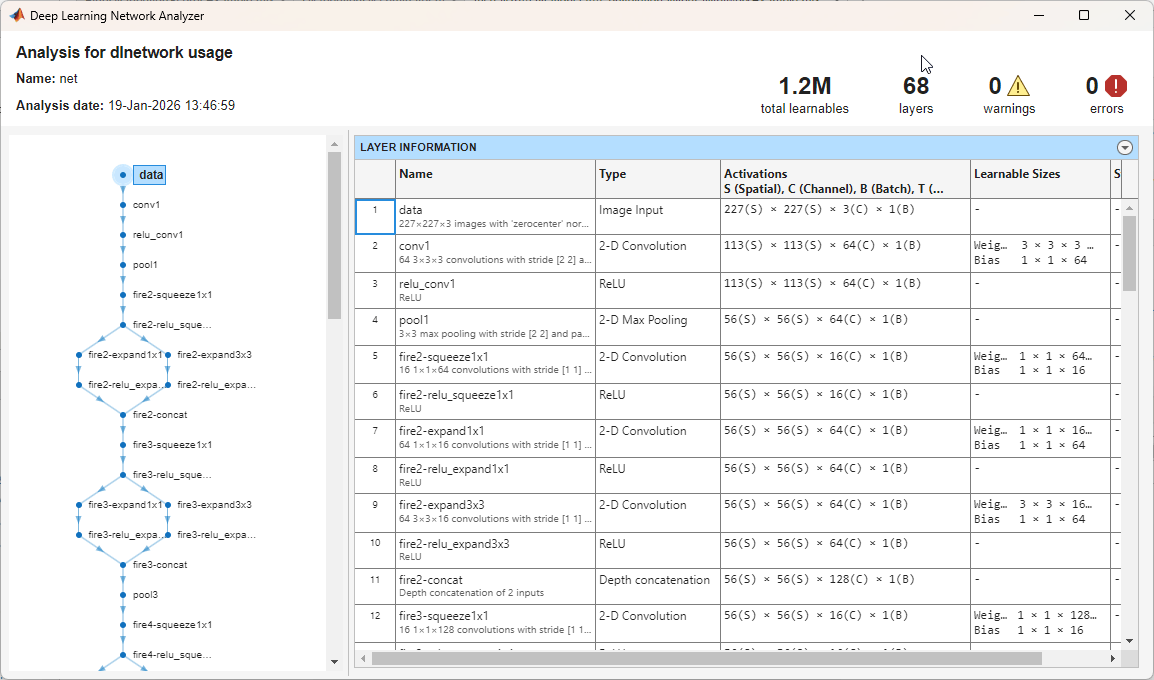The Image Input layer specifies the input size. You can resize the image before passing it through the network, but the network also can process larger images. If you feed the network larger images, the activations also become larger. However, since the network is trained on images of size 227-by-227, it is not trained to recognize objects or features larger than that size.

### Show Activations of First Convolutional Layer

Investigate features by observing which areas in the convolutional layers activate on an image and comparing with the corresponding areas in the original images. Each layer of a convolutional neural network consists of many 2-D arrays called channels. Pass the image through the network and examine the output activations of the `conv1` layer.

`act1 = activations(net,im,'conv1');`

The activations are returned as a 3-D array, with the third dimension indexing the channel on the `conv1` layer. To show these activations using the `imtile` function, reshape the array to 4-D. The third dimension in the input to `imtile` represents the image color. Set the third dimension to have size 1 because the activations do not have color. The fourth dimension indexes the channel.

```sz = size(act1); act1 = reshape(act1,[sz(1) sz(2) 1 sz(3)]);```

Now you can show the activations. Each activation can take any value, so normalize the output using `mat2gray`. All activations are scaled so that the minimum activation is 0 and the maximum is 1. Display the 64 images on an 8-by-8 grid, one for each channel in the layer.

```I = imtile(mat2gray(act1),'GridSize',[8 8]); imshow(I)```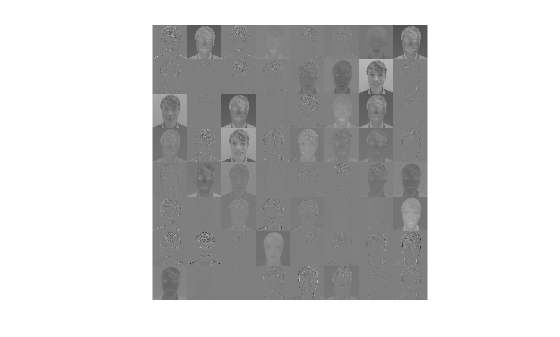### Investigate the Activations in Specific Channels

Each tile in the grid of activations is the output of a channel in the `conv1` layer. White pixels represent strong positive activations and black pixels represent strong negative activations. A channel that is mostly gray does not activate as strongly on the input image. The position of a pixel in the activation of a channel corresponds to the same position in the original image. A white pixel at some location in a channel indicates that the channel is strongly activated at that position.

Resize the activations in channel 22 to have the same size as the original image and display the activations.

```act1ch22 = act1(:,:,:,22); act1ch22 = mat2gray(act1ch22); act1ch22 = imresize(act1ch22,imgSize); I = imtile({im,act1ch22}); imshow(I)```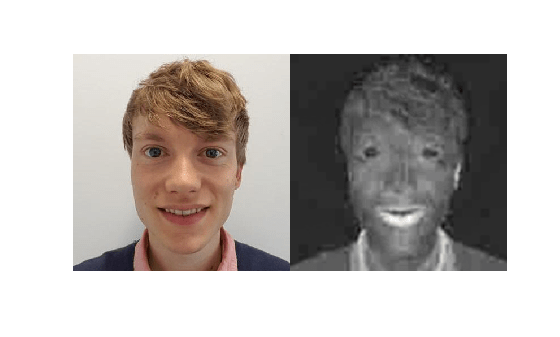You can see that this channel activates on red pixels, because the whiter pixels in the channel correspond to red areas in the original image.

### Find the Strongest Activation Channel

You also can try to find interesting channels by programmatically investigating channels with large activations. Find the channel with the largest activation using the `max` function, resize, and show the activations.

```[maxValue,maxValueIndex] = max(max(max(act1))); act1chMax = act1(:,:,:,maxValueIndex); act1chMax = mat2gray(act1chMax); act1chMax = imresize(act1chMax,imgSize); I = imtile({im,act1chMax}); imshow(I)```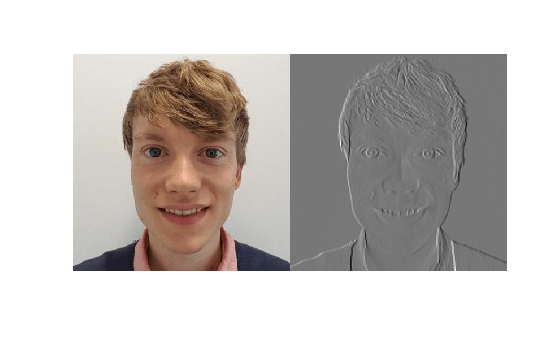Compare to the original image and notice that this channel activates on edges. It activates positively on light left/dark right edges, and negatively on dark left/light right edges.

### Investigate a Deeper Layer

Most convolutional neural networks learn to detect features like color and edges in their first convolutional layer. In deeper convolutional layers, the network learns to detect more complicated features. Later layers build up their features by combining features of earlier layers. Investigate the `fire6-squeeze1x1` layer in the same way as the `conv1` layer. Calculate, reshape, and show the activations in a grid.

```act6 = activations(net,im,'fire6-squeeze1x1'); sz = size(act6); act6 = reshape(act6,[sz(1) sz(2) 1 sz(3)]); I = imtile(imresize(mat2gray(act6),[64 64]),'GridSize',[6 8]); imshow(I)```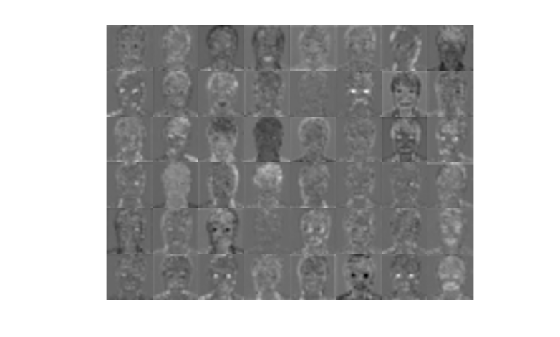There are too many images to investigate in detail, so focus on some of the more interesting ones. Display the strongest activation in the `fire6-squeeze1x1` layer.

```[maxValue6,maxValueIndex6] = max(max(max(act6))); act6chMax = act6(:,:,:,maxValueIndex6); imshow(imresize(mat2gray(act6chMax),imgSize))```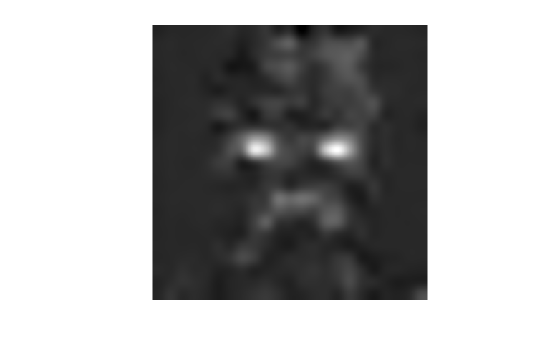In this case, the maximum activation channel is not as interesting for detailed features as some others, and shows strong negative (dark) as well as positive (light) activation. This channel is possibly focusing on faces.

In the grid of all channels, there are channels that might be activating on eyes. Investigate channels 14 and 47 further.

```I = imtile(imresize(mat2gray(act6(:,:,:,[14 47])),imgSize)); imshow(I)```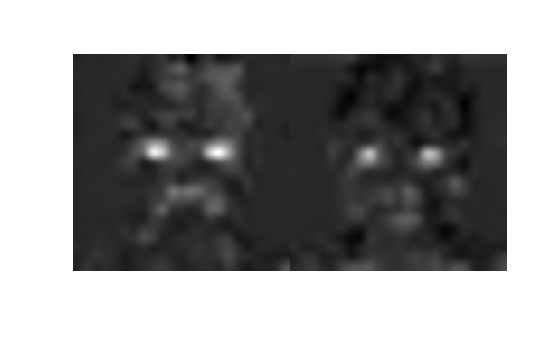Many of the channels contain areas of activation that are both light and dark. These are positive and negative activations, respectively. However, only the positive activations are used because of the rectified linear unit (ReLU) that follows the `fire6-squeeze1x1` layer. To investigate only positive activations, repeat the analysis to visualize the activations of the `fire6-relu_squeeze1x1` layer.

```act6relu = activations(net,im,'fire6-relu_squeeze1x1'); sz = size(act6relu); act6relu = reshape(act6relu,[sz(1) sz(2) 1 sz(3)]); I = imtile(imresize(mat2gray(act6relu(:,:,:,[14 47])),imgSize)); imshow(I)```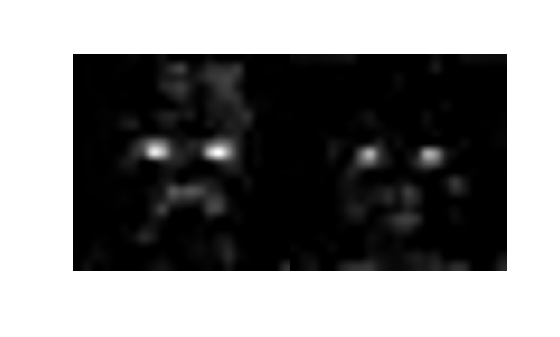Compared to the activations of the `fire6-squeeze1x1` layer, the activations of the `fire6-relu_squeeze1x1` layer clearly pinpoint areas of the image that have strong facial features.

### Test Whether a Channel Recognizes Eyes

Check whether channels 14 and 47 of the `fire6-relu_squeeze1x1` layer activate on eyes. Input a new image with one closed eye to the network and compare the resulting activations with the activations of the original image.

Read and show the image with one closed eye and compute the activations of the `fire6-relu_squeeze1x1` layer.

```imClosed = imread('face-eye-closed.jpg'); imshow(imClosed)```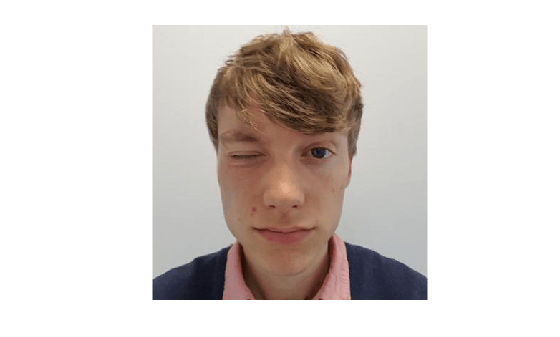```act6Closed = activations(net,imClosed,'fire6-relu_squeeze1x1'); sz = size(act6Closed); act6Closed = reshape(act6Closed,[sz(1),sz(2),1,sz(3)]);```

Plot the images and activations in one figure.

```channelsClosed = repmat(imresize(mat2gray(act6Closed(:,:,:,[14 47])),imgSize),[1 1 3]); channelsOpen = repmat(imresize(mat2gray(act6relu(:,:,:,[14 47])),imgSize),[1 1 3]); I = imtile(cat(4,im,channelsOpen*255,imClosed,channelsClosed*255)); imshow(I) title('Input Image, Channel 14, Channel 47');```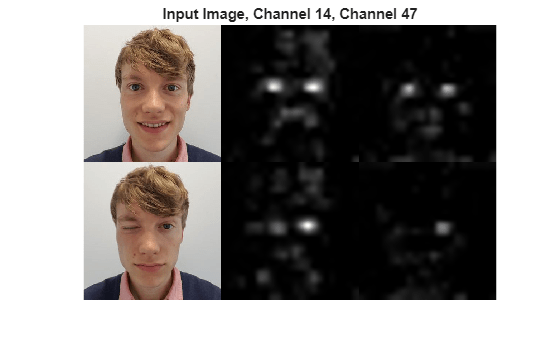You can see from the activations that both channels 14 and 47 activate on individual eyes, and to some degree also on the area around the mouth.

The network has never been told to learn about eyes, but it has learned that eyes are a useful feature to distinguish between classes of images. Previous machine learning approaches often manually designed features specific to the problem, but these deep convolutional networks can learn useful features for themselves. For example, learning to identify eyes could help the network distinguish between a leopard and a leopard print rug.

Download ebook